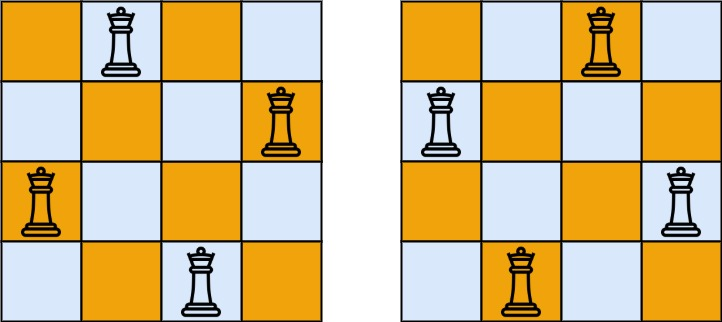# 52. N-Queens II | LeetCode Solution

## 52. N-Queens II | LeetCode Solution

The n-queens puzzle is the problem of placing `n` queens on an `n x n` chessboard such that no two queens attack each other.

Given an integer `n`, return the number of distinct solutions to the n-queens puzzle.

Example 1:```Input: n = 4
Output: 2
Explanation: There are two distinct solutions to the 4-queens puzzle as shown.
```

Example 2:

```Input: n = 1
Output: 1
```

Constraints:

• `1 <= n <= 9`

class Solution {
public:
int ans=0;
bool check(int n,int r,int c,vector<string>&v){

for(int i=0; i<r; i++){
if(v[i][c]=='Q')return false;
}
for(int i=r-1,j=c-1; i>=0 && j>=0; i--,j--){
if(v[i][j]=='Q')return false;
}
for(int i=r-1,j=c+1; i>=0 && c<n; i--,j++){
if(v[i][j]=='Q')return false;
}

return true;
}

void helper(int n,int r,int c,vector<string>&v){
if(r>=n){
ans++;
return;
}
for(int i=0; i<n; i++){
if(check(n,r,i,v)){
v[r][i]='Q';
helper(n,r+1,0,v);
v[r][i]='.';
}
}
}

int totalNQueens(int n) {
vector<string>v(n,string(n,'.'));
helper(n,0,0,v);
return ans;
}
};